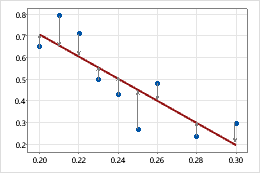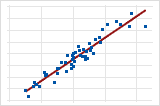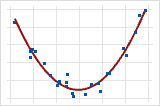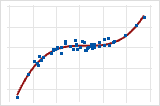# Fitted regression lines

A fitted regression line on a graph represents of the mathematical regression equation for your data.

Fitted regression lines are drawn using the least squares estimation method which minimizes the sum of the squared distances between the points and the fitted line.Use fitted regression lines to illustrate the relationship between a predictor variable (x) and a response variable (y) and to evaluate whether a linear, quadratic, or cubic regression fits your data.

Linear
A linear model can show a steady rate of increase or decrease in the data.A quadratic model (often approximately in the shape of a U or an inverted U) can explain curvature in the data.Cubic
A cubic model can describe a "peak-and-valley" pattern in the data.### Example of using fitted regression lines to determine the model

You are studying the relationship between a particular machine setting and the amount of energy consumed. You suspect that this relationship has curvature, but you need to confirm and document the curvature. You produce the following plots. The quadratic model appears to fit the data better than a linear model.

## Add a fitted regression line when you create a graph

You can add fitted regression lines when you create a graph, such as a scatterplot or a matrix plot.

1. In the dialog box for the graph you are creating, click Data View.
2. Click the Regression tab.
3. Under Model Order, select the model that fits your data.
4. To fit the regression line without the y-intercept, deselect Fit intercept. By default, Minitab includes a term for the y-intercept. Usually, you should include the intercept in the model.

## Add a fitted regression line to an existing graph

You can add fitted regression lines to an existing graph, such as a scatterplot or a matrix plot.

1. Double-click the graph.
2. Right-click the graph and choose Add > Regression Fit.
3. Under Model Order, select the model that fits your data.
4. To fit the regression line without the y-intercept, deselect Fit intercept. By default, Minitab includes a term for the y-intercept. Usually, you should include the intercept in the model.

## Edit fitted regression lines

After you add a fitted regression line, you can edit it.

1. Double-click the graph.
2. Double-click the fitted regression line. For more information on selecting lines, go to Select groups and single items on a graph.
3. Select the tab to make the edits that you want.
• Attributes: Change the color, size, or line type.
• Groups: Enter a categorical variable for grouping.
• Options: Select the model that fits your data.
By using this site you agree to the use of cookies for analytics and personalized content.  Read our policy